# GSEB Solutions Class 10 Maths Chapter 2 Polynomials Ex 2.1

Gujarat Board GSEB Solutions Class 10 Maths Chapter 2 Polynomials Ex 2.1 Textbook Questions and Answers.

## Gujarat Board Textbook Solutions Class 10 Maths Chapter 2 Polynomials Ex 2.1

Question 1.
The graphs of y = p(x) are given in figures, for some polynomials p(x). Find the number of zeroes of p(x), in each case.
1.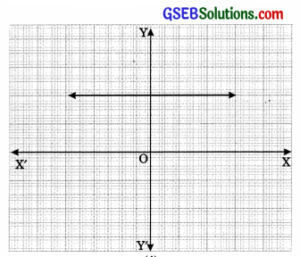2.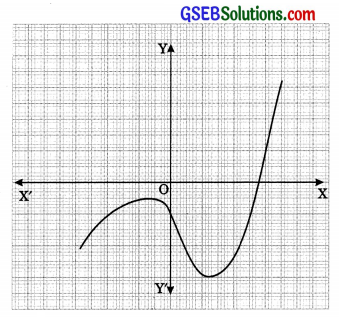3.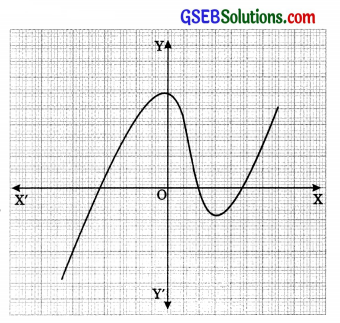4.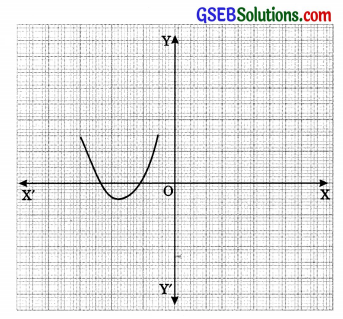5.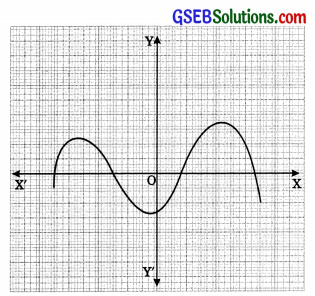6.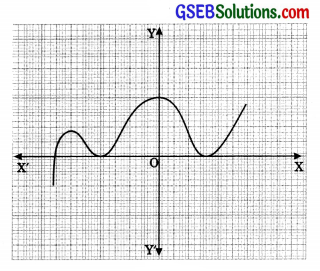Solution:

1. Graph does not intersect at any point on x-axis, hence there is no zeroes.
2. Graph intersect at one point on the x-axis, hence the number of zeroes is 1.
3. Graph intersect at three points on the x-axis, hence the number of zeroes is 3.
4. Graph intersect at two points on the x-axis, hence the number of zeroes is 2.
5. Graph intersect at four points on the x-axis, hence the number of zeroes is 4.
6. Graph intersect at three points on the x-axis, hence the number of zeroes is 3.